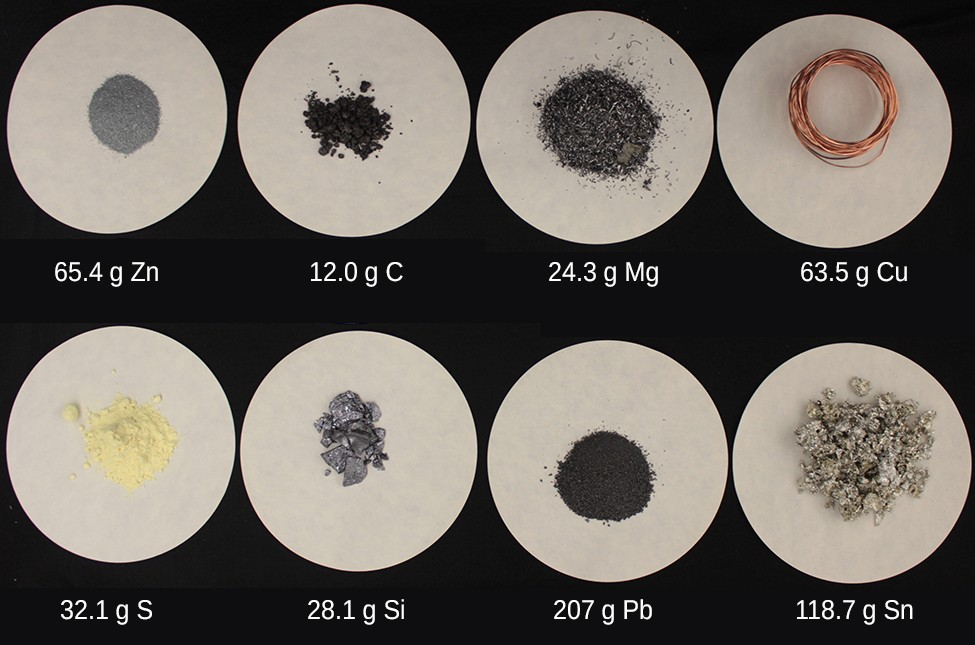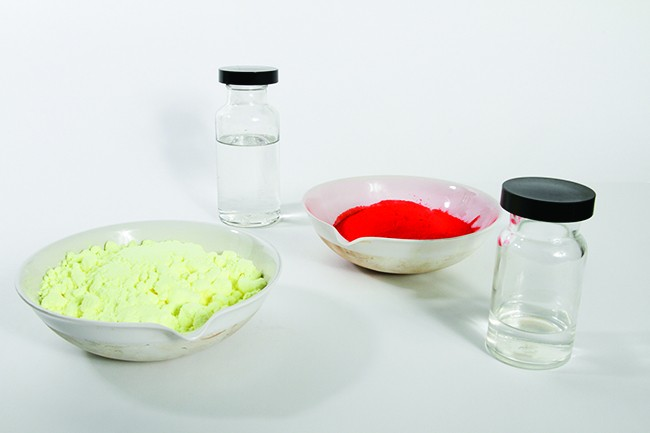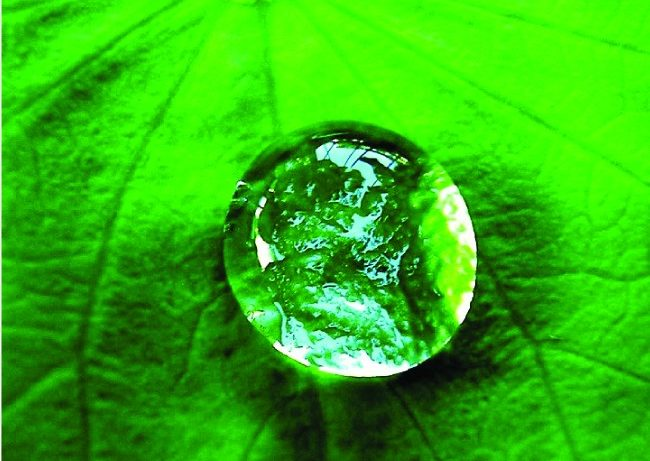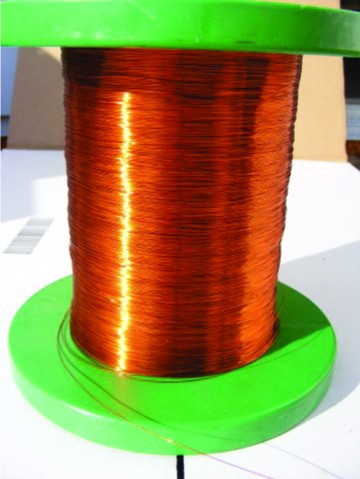## 7.1 The Mole Concept

### Learning Objectives

By the end of this section, you will be able to:

• Define the amount unit mole and the related quantity Avogadro’s number
• Explain the relation between mass, moles, and numbers of atoms or molecules, and perform calculations deriving these quantities from one another

## The Mole

The identity of a substance is defined not only by the types of atoms or ions it contains, but by the quantity of each type of atom or ion. For example, water, H2O, and hydrogen peroxide, H2O2, are alike in that their respective molecules are composed of hydrogen and oxygen atoms. However, because a hydrogen peroxide molecule contains two oxygen atoms, as opposed to the water molecule, which has only one, the two substances exhibit very different properties. Today, we possess sophisticated instruments that allow the direct measurement of these defining microscopic traits; however, the same traits were originally derived from the measurement of macroscopic properties (the masses and volumes of bulk quantities of matter) using relatively simple tools (balances and volumetric glassware). This experimental approach required the introduction of a new unit for amount of substances, the mole, which remains indispensable in modern chemical science.

The mole is an amount unit similar to familiar units like pair, dozen, gross, etc. It provides a specific measure of the number of atoms or molecules in a bulk sample of matter. A mole is defined as the amount of substance containing the same number of discrete entities (atoms, molecules, ions, etc.) as the number of atoms in a sample of pure 12C weighing exactly 12 g. One Latin connotation for the word “mole” is “large mass” or “bulk,” which is consistent with its use as the name for this unit. The mole provides a link between an easily measured macroscopic property, bulk mass, and an extremely important fundamental property, number of atoms, molecules, and so forth.

The number of entities composing a mole has been experimentally determined to be $6.02214179\times {10}^{23}$, a fundamental constant named Avogadro’s number (NA) or the Avogadro constant in honor of Italian scientist Amedeo Avogadro. This constant is properly reported with an explicit unit of “per mole,” a conveniently rounded version being $6.022\times {10}^{23}\text{/mol}$.

Consistent with its definition as an amount unit, 1 mole of any element contains the same number of atoms as 1 mole of any other element. The masses of 1 mole of different elements, however, are different, since the masses of the individual atoms are drastically different. The molar mass of an element (or compound) is the mass in grams of 1 mole of that substance, a property expressed in units of grams per mole (g/mol) (see Figure 1).Figure 1. Each sample contains 6.022 × 1023 atoms—1.00 mol of atoms. From left to right (top row): 65.4g zinc, 12.0g carbon, 24.3g magnesium, and 63.5g copper. From left to right (bottom row): 32.1g sulfur, 28.1g silicon, 207g lead, and 118.7g tin. (credit: modification of work by Mark Ott)

Because the definitions of both the mole and the atomic mass unit are based on the same reference substance, 12C, the molar mass of any substance is numerically equivalent to its atomic or formula weight in amu. Per the amu definition, a single 12C atom weighs 12 amu (its atomic mass is 12 amu). According to the definition of the mole, 12 g of 12C contains 1 mole of 12C atoms (its molar mass is 12 g/mol). This relationship holds for all elements, since their atomic masses are measured relative to that of the amu-reference substance, 12C. Extending this principle, the molar mass of a compound in grams is likewise numerically equivalent to its formula mass in amu (Figure 2).Figure 2. Each sample contains 6.02 × 1023 molecules or formula units—1.00 mol of the compound or element. Clock-wise from the upper left: 130.2g of C8H17OH (1-octanol, formula mass 130.2 amu), 454.9g of HgI2 (mercury(II) iodide, formula mass 459.9 amu), 32.0g of CH3OH (methanol, formula mass 32.0 amu) and 256.5g of S8 (sulfur, formula mass 256.6 amu). (credit: Sahar Atwa)

Element Average Atomic Mass (amu) Molar Mass (g/mol) Atoms/Mole
C 12.01 12.01 $6.022\times {10}^{23}$
H 1.008 1.008 $6.022\times {10}^{23}$
O 16.00 16.00 $6.022\times {10}^{23}$
Na 22.99 22.99 $6.022\times {10}^{23}$
Cl 33.45 33.45 $6.022\times {10}^{23}$Figure 3. A single drop of water.

While atomic mass and molar mass are numerically equivalent, keep in mind that they are vastly different in terms of scale, as represented by the vast difference in the magnitudes of their respective units (amu versus g). To appreciate the enormity of the mole, consider a small drop of water weighing about 0.03 g (see Figure 3). The number of molecules in a single droplet of water is roughly 100 billion times greater than the number of people on earth.

Although this represents just a tiny fraction of 1 mole of water (~18 g), it contains more water molecules than can be clearly imagined. If the molecules were distributed equally among the roughly seven billion people on earth, each person would receive more than 100 billion molecules.

The mole is used in chemistry to represent $6.022\times {10}^{23}$ of something, but it can be difficult to conceptualize such a large number. Watch this video to learn more.

The relationships between formula mass, the mole, and Avogadro’s number can be applied to compute various quantities that describe the composition of substances and compounds. For example, if we know the mass and chemical composition of a substance, we can determine the number of moles and calculate number of atoms or molecules in the sample. Likewise, if we know the number of moles of a substance, we can derive the number of atoms or molecules and calculate the substance’s mass.

### Example 1: Deriving Moles from Grams for an Element

According to nutritional guidelines from the US Department of Agriculture, the estimated average requirement for dietary potassium is 4.7 g. What is the estimated average requirement of potassium in moles?

Beryllium is a light metal used to fabricate transparent X-ray windows for medical imaging instruments. How many moles of Be are in a thin-foil window weighing 3.24 g?

### Example 2: Deriving Grams from Moles for an Element

A liter of air contains $9.2\times {10}^{-4}$ mol argon. What is the mass of Ar in a liter of air?

What is the mass of 2.561 mol of gold?

### Example 3: Deriving Number of Atoms from Mass for an Element

Copper is commonly used to fabricate electrical wire (Figure 7). How many copper atoms are in 5.00 g of copper wire?Figure 7. Copper wire is composed of many, many atoms of Cu. (credit: Emilian Robert Vicol)

A prospector panning for gold in a river collects 15.00 g of pure gold. How many Au atoms are in this quantity of gold?

### Example 4: Deriving Moles from Grams for a Compound

Our bodies synthesize protein from amino acids. One of these amino acids is glycine, which has the molecular formula C2H5O2N. How many moles of glycine molecules are contained in 28.35 g of glycine?

How many moles of sucrose, C12H22O11, are in a 25-g sample of sucrose?

### Example 5: Deriving Grams from Moles for a Compound

Vitamin C is a covalent compound with the molecular formula C6H8O6. The recommended daily dietary allowance of vitamin C for children aged 4–8 years is $1.42\times {10}^{-4}\text{mol.}$ What is the mass of this allowance in grams?

What is the mass of 0.443 mol of hydrazine, N2H4?

### Example 6: Deriving the Number of Atoms and Molecules from the Mass of a Compound

A packet of an artificial sweetener contains 0.0400 g of saccharin (C7H5NO3S). (a) Given that saccharin has a molar mass of 183.18 g/mol, how many saccharin molecules are in a 0.0400-g sample of saccharin?

How many C4H10 molecules are contained in 9.213 g of this compound?

## Chemical Formulas and the Mole

Suppose we want to know the number of hydrogen atoms found in a dozen CH4 molecule. For each CH4 molecule there are four hydrogen atoms, we determine this from the chemical formula, there is one carbon atom per one CH4 molecule. As shown below, if we have one dozen molecules of CH4 we can determine that we will have a total of 48 hydrogen’s using dimensional analysis.

$12\text{ molecules CH}_{4}\left(\frac{\text{4 }\text{atoms H}}{\text{1 molecule CH}_{4}}\right)=48\text{ }\text{atoms H}$

Since is unlikely a chemist will be working with a single molecule, and much more likely to be working molecules on the scale of Avogadro’s number, it makes more sense for us to relate the number of moles of H to the moles of CH4.   If we had 12 mole sample of CH4, how many moles of H atoms will be present in the sample?  We can derive a mole to mole relationship between H and CH4 using the chemical formula.

$\left(\frac{\text{4 }\text{mol H}}{\text{1 mol CH}_{4}}\right)$

Therefore, if we have a 12 mol sample of CH4, we can calculate the moles of H present in the sample:

$\text{12 }\cancel{\text{mol CH}_{4}}\left(\frac{\text{4 }\text{mol H}}{\text{1 }\cancel{\text{mol CH}_{4}}}\right)=48 \text{ mol H}$

All we need to derive a mol to mol relationship between a compound and an element within that compound is the chemical formula.

### Example 7: Converting between moles of a compound and moles of an element within the compound

An organic compound, commonly known as strawberry aldehyde, is used in the flavor industry in artificial fruit flavors. Given the formula C12H14O3, determine the moles of each element within 2.50 moles of strawberry aldehyde?

How many moles of nitrogen are in 10.0 moles of N2O4?

We can use mol to mol ratios in combination with other mole conversions, such as Avogadro’s number and molar mass.

### Example 8: Converting between grams of a compound and grams of an element within the compound

Isoamyl acetate, C7H14O2, is an organic ester commonly referred to as banana oil due to it strong banana odor.  How many grams of carbon will be in a 0.500 g sample of isoamyl acetate?

How many grams of oxygen are in 5.0 g of N2O4?

### Example 9: Converting between grams of a compound and atoms of an element within the compound

Cow’s and other livestock are responsible for nearly 40% of global methane (CH4) emissions.  A cow produces on average, 260.0 g of methane per day.  How many atoms of hydrogen is present in a 260.0 g sample of methane?

How many atoms of carbon is present in a 260.0 g sample of methane?

### Key Concepts and Summary

A convenient amount unit for expressing very large numbers of atoms or molecules is the mole. Experimental measurements have determined the number of entities composing 1 mole of substance to be $6.022\times {10}^{23}$, a quantity called Avogadro’s number. The mass in grams of 1 mole of substance is its molar mass. Due to the use of the same reference substance in defining the atomic mass unit and the mole, the formula mass (amu) and molar mass (g/mol) for any substance are numerically equivalent (for example, one H2O molecule weighs approximately18 amu and 1 mole of H2O molecules weighs approximately 18 g).

### Exercises

1. Write a sentence that describes how to determine the number of moles of a compound in a known mass of the compound if we know its molecular formula.
2. Compare 1 mole of H2, 1 mole of O2, and 1 mole of F2.
1. Which has the largest number of molecules? Explain why.
2. Which has the greatest mass? Explain why.
3. Determine the number of indicated particles in the following;
1. number of atoms of F in 1.5 moles of F
2. number of atoms of Al in 5.2 mol Al
3. number of molecules of CO2 in 0.67 mol of CO2
4. number of molecules of C2H5OH in 0.0250 mol of C2H5OH
5. number of formula units of NaCl in 0.050 mol of NaCl
6. number of formula units of Ca3(PO4)2 in 3.40 mol of Ca3(PO4)2
4. Calculate the molar mass of each of the following compounds:
1. HF
2. NH3
3. HNO3
4. Ag2SO4
5. B(OH)3
6. S8
7. C5H12
8. Sc2(SO4)3
9. CH3COCH3 (acetone)
10. C6H12O6 (glucose)
5. Determine the number of moles in the following;
1. number of moles of Cu in 5.50 g Cu
2. number of moles of S in 30.2 g S
3. number of moles of CCl4 in 0.250 g CCl4
4. number of moles of C12H22O11 in 100.0 g C12H22O11
5. number of moles of Na2S in 12.0 g Na2S
6. number of moles of Ca3(PO4)2 in 20.0 g Ca3(PO4)2
6. Which contains the greatest mass of oxygen: 0.75 mol of ethanol (C2H5OH), 0.60 mol of formic acid (HCO2H), or 1.0 mol of water (H2O)? Explain why.
7. Which contains the greatest number of moles of oxygen atoms: 1 mol of ethanol (C2H5OH), 1 mol of formic acid (HCO2H), or 1 mol of water (H2O)? Explain why.
8. Determine the number of moles of compound and the number of moles of each type of atom in each of the following:
1. 25.0 g of propylene, C3H6
2. $3.06\times {10}^{-3}\text{g}$ of the amino acid glycine, C2H5NO2
3. 25 lb of the herbicide Treflan, C13H16N2O4F (1 lb = 454 g)
4. 0.125 kg of the insecticide Paris Green, Cu4(AsO3)2(CH3CO2)2
5. 325 mg of aspirin, C6H4(CO2H)(CO2CH3)
9. Determine the number of moles of the compound and determine the number of moles of each type of atom in each of the following:
1. 2.12 g of potassium bromide, KBr
2. 0.1488 g of phosphoric acid, H3PO4
3. 23 kg of calcium carbonate, CaCO3
4. 78.452 g of aluminum sulfate, Al2(SO4)3
5. 0.1250 mg of caffeine, C8H10N4O2
10. The approximate minimum daily dietary requirement of the amino acid leucine, C6H13NO2, is 1.1 g. What is this requirement in moles?
11. Determine the mass in grams of each of the following:
1. 0.600 mol of oxygen atoms
2. 0.600 mol of oxygen molecules, O2
3. 0.600 mol of ozone molecules, O3
12. A 55-kg woman has $7.5\times {10}^{-3}\text{mol}$ of hemoglobin (molar mass = 64,456 g/mol) in her blood. How many hemoglobin molecules is this? What is this quantity in grams?
13. Determine the number of atoms and the mass of zirconium, silicon, and oxygen found in 0.3384 mol of zircon, ZrSiO4, a semiprecious stone.
14. Determine which of the following contains the greatest mass of hydrogen: 1 mol of CH4, 0.6 mol of C6H6, or 0.4 mol of C3H8.
15. Determine which of the following contains the greatest mass of aluminum: 122 g of AlPO4, 266 g of Al2Cl6, or 225 g of Al2S3.
16. Diamond is one form of elemental carbon. An engagement ring contains a diamond weighing 1.25 carats (1 carat = 200 mg). How many atoms are present in the diamond?
17. The Cullinan diamond was the largest natural diamond ever found (January 25, 1905). It weighed 3104 carats (1 carat = 200 mg). How many carbon atoms were present in the stone
18. One 55-gram serving of a particular cereal supplies 270 mg of sodium, 11% of the recommended daily allowance. How many moles and atoms of sodium are in the recommended daily allowance?
19. A certain nut crunch cereal contains 11.0 grams of sugar (sucrose, C12H22O11) per serving size of 60.0 grams. How many servings of this cereal must be eaten to consume 0.0278 moles of sugar?
20. A tube of toothpaste contains 0.76 g of sodium monofluorophosphate (Na2PO3F) in 100 mL
1. What mass of fluorine atoms in mg was present?
2. How many fluorine atoms were present?
21. Which of the following represents the least number of molecules?
1. 20.0 g of H2O (18.02 g/mol)
2. 77.0 g of CH4 (16.06 g/mol)
3. 68.0 g of CaH2 (42.09 g/mol)
4. 100.0 g of N2O (44.02 g/mol)
5. 84.0 g of HF (20.01 g/mol)

## Glossary

Avogadro’s number (NA): experimentally determined value of the number of entities comprising 1 mole of substance, equal to $6.022\times {10}^{23}{\text{mol}}^{-1}$

molar mass: mass in grams of 1 mole of a substance

mole: amount of substance containing the same number of atoms, molecules, ions, or other entities as the number of atoms in exactly 12 grams of 12C